Скачать презентацию Regression with a Binary Dependent Variable Linear

6f385c157f43a8f5bec7f03ae7dcbef8.ppt

• Количество слайдов: 61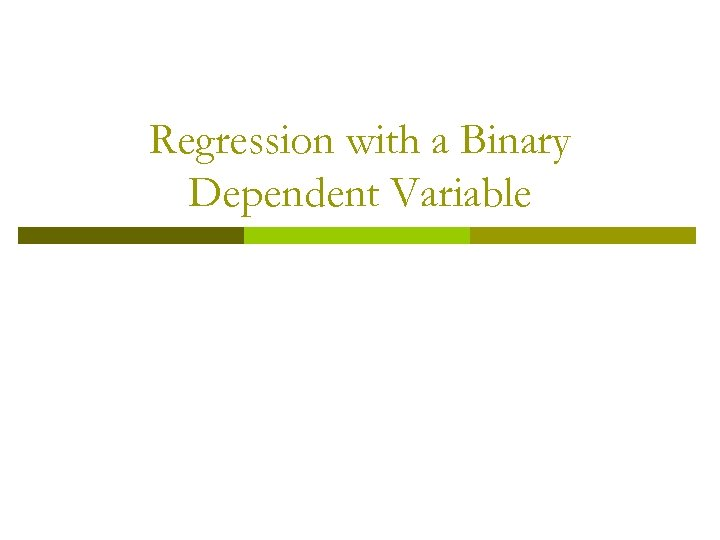Regression with a Binary Dependent Variable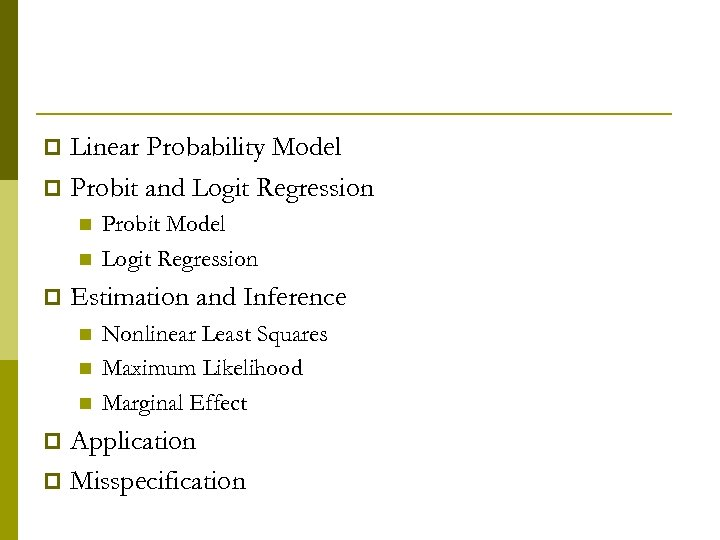Linear Probability Model p Probit and Logit Regression p n n p Probit Model Logit Regression Estimation and Inference n n n Nonlinear Least Squares Maximum Likelihood Marginal Effect Application p Misspecification p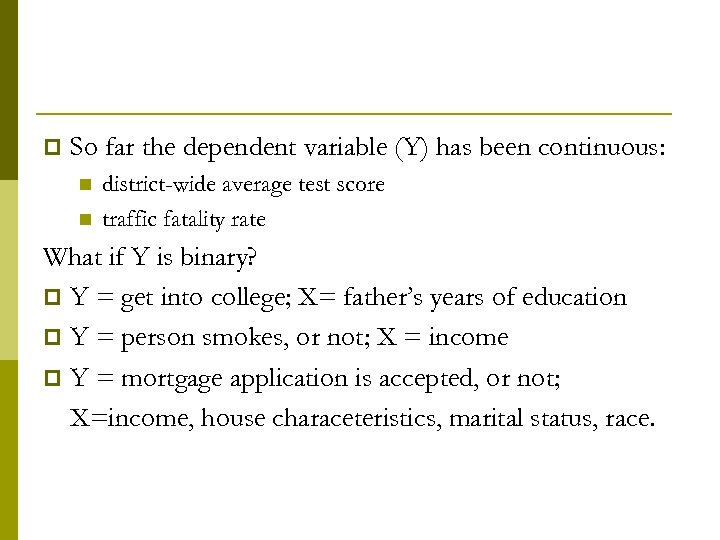p So far the dependent variable (Y) has been continuous: n n district-wide average test score traffic fatality rate What if Y is binary? p Y = get into college; X= father’s years of education p Y = person smokes, or not; X = income p Y = mortgage application is accepted, or not; X=income, house characeteristics, marital status, race.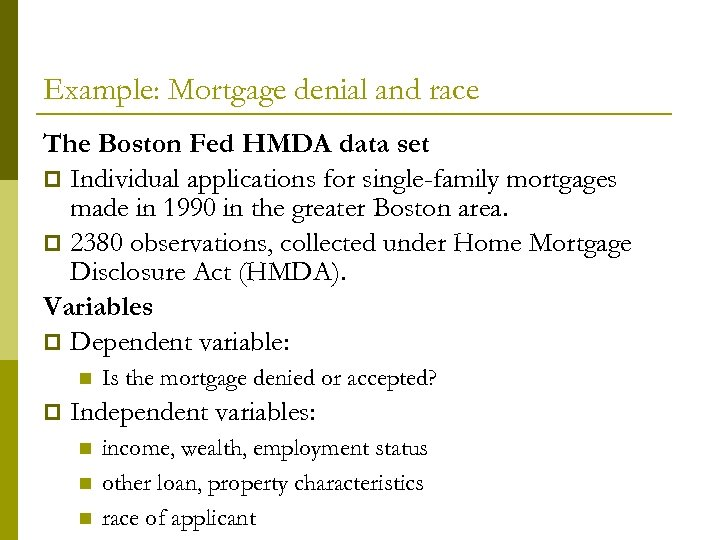Example: Mortgage denial and race The Boston Fed HMDA data set p Individual applications for single-family mortgages made in 1990 in the greater Boston area. p 2380 observations, collected under Home Mortgage Disclosure Act (HMDA). Variables p Dependent variable: n p Is the mortgage denied or accepted? Independent variables: n n n income, wealth, employment status other loan, property characteristics race of applicant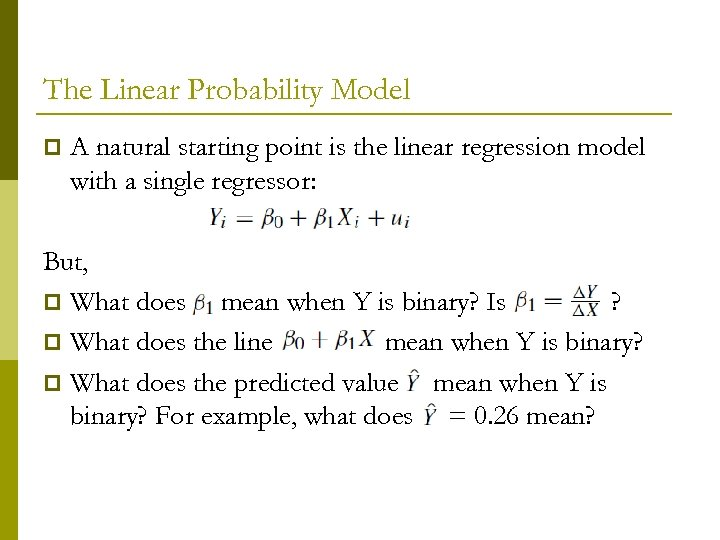The Linear Probability Model p A natural starting point is the linear regression model with a single regressor: But, p What does mean when Y is binary? Is ? p What does the line mean when Y is binary? p What does the predicted value mean when Y is binary? For example, what does = 0. 26 mean?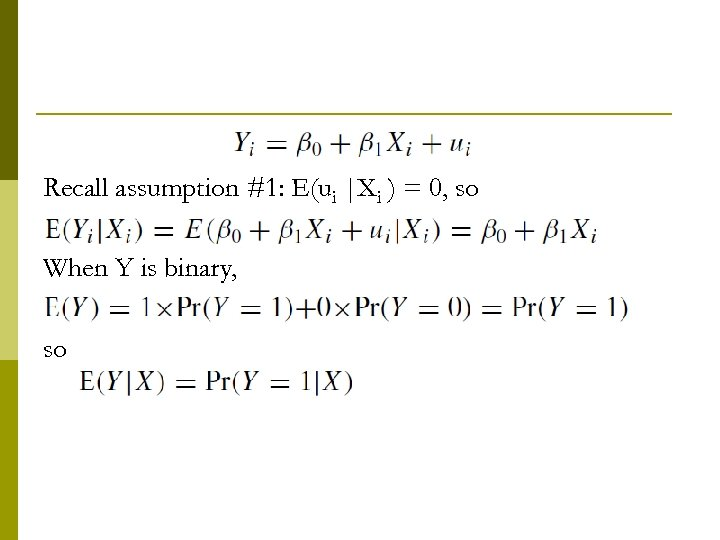Recall assumption #1: E(ui |Xi ) = 0, so When Y is binary, so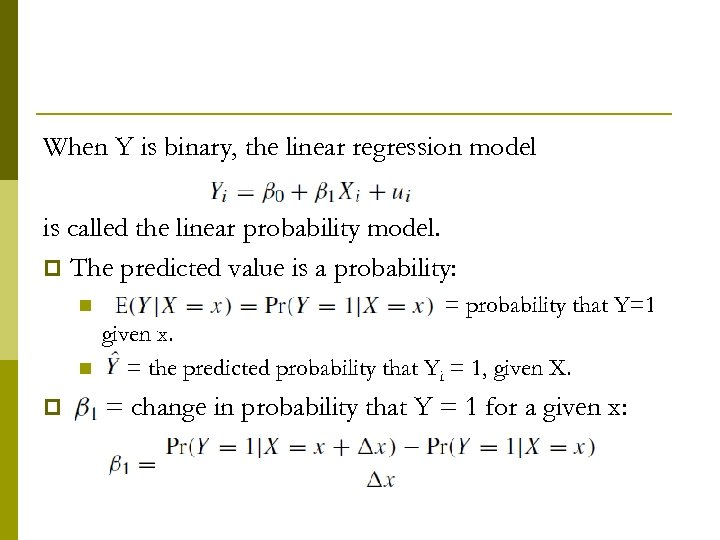When Y is binary, the linear regression model is called the linear probability model. p The predicted value is a probability: n n p = probability that Y=1 given x. = the predicted probability that Yi = 1, given X. = change in probability that Y = 1 for a given x: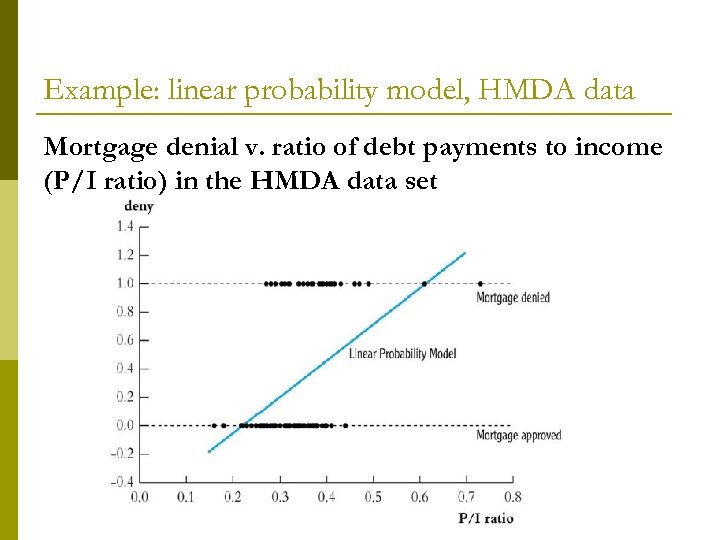Example: linear probability model, HMDA data Mortgage denial v. ratio of debt payments to income (P/I ratio) in the HMDA data set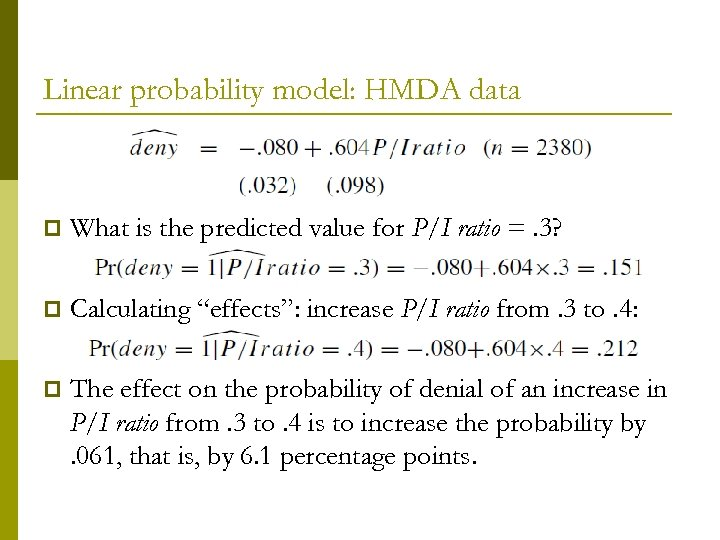Linear probability model: HMDA data p What is the predicted value for P/I ratio =. 3? p Calculating “effects”: increase P/I ratio from. 3 to. 4: p The effect on the probability of denial of an increase in P/I ratio from. 3 to. 4 is to increase the probability by. 061, that is, by 6. 1 percentage points.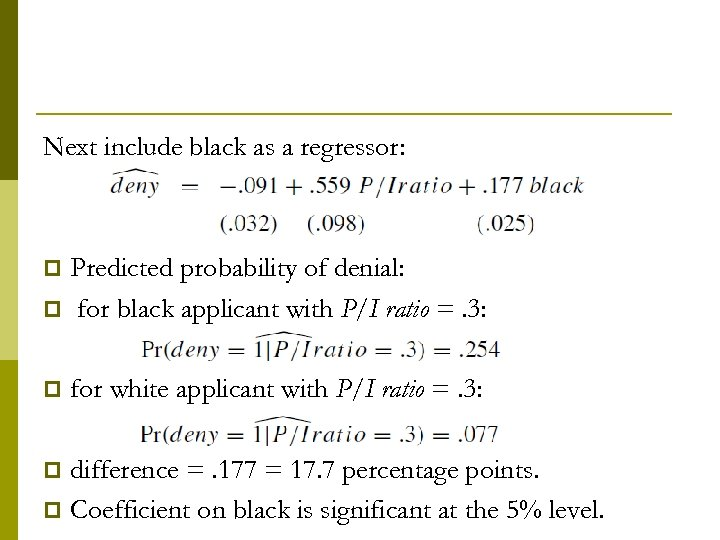Next include black as a regressor: Predicted probability of denial: p for black applicant with P/I ratio =. 3: p p for white applicant with P/I ratio =. 3: difference =. 177 = 17. 7 percentage points. p Coefficient on black is significant at the 5% level. p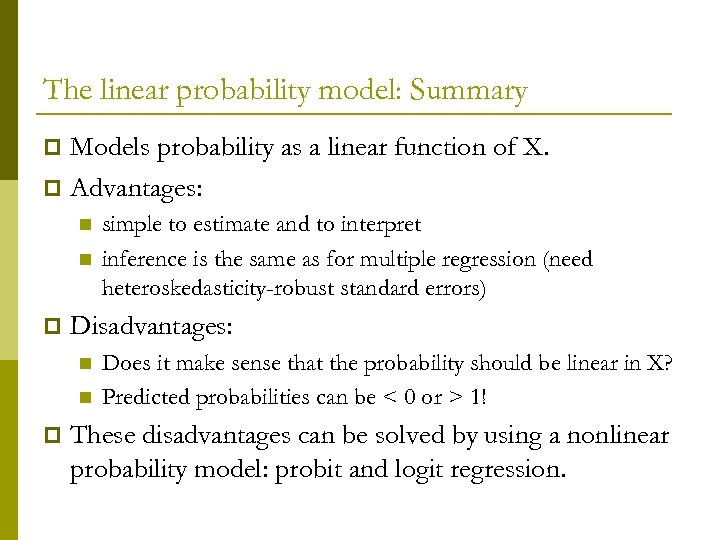The linear probability model: Summary Models probability as a linear function of X. p Advantages: p n n p Disadvantages: n n p simple to estimate and to interpret inference is the same as for multiple regression (need heteroskedasticity-robust standard errors) Does it make sense that the probability should be linear in X? Predicted probabilities can be < 0 or > 1! These disadvantages can be solved by using a nonlinear probability model: probit and logit regression.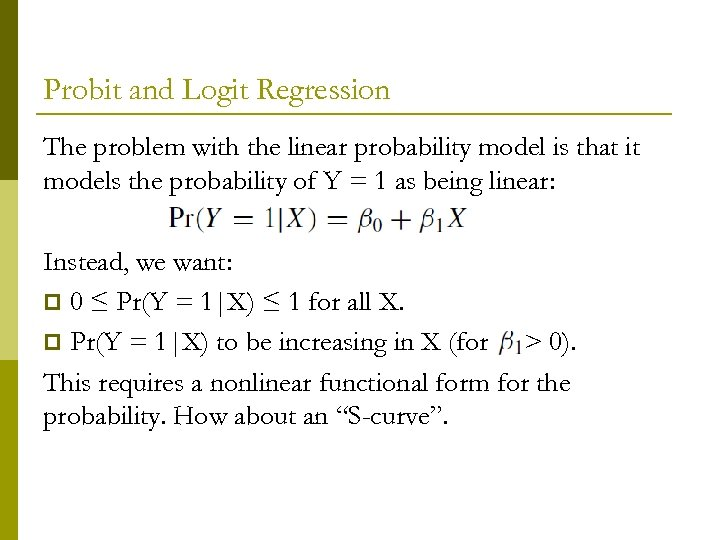Probit and Logit Regression The problem with the linear probability model is that it models the probability of Y = 1 as being linear: Instead, we want: p 0 ≤ Pr(Y = 1|X) ≤ 1 for all X. p Pr(Y = 1|X) to be increasing in X (for > 0). This requires a nonlinear functional form for the probability. How about an “S-curve”.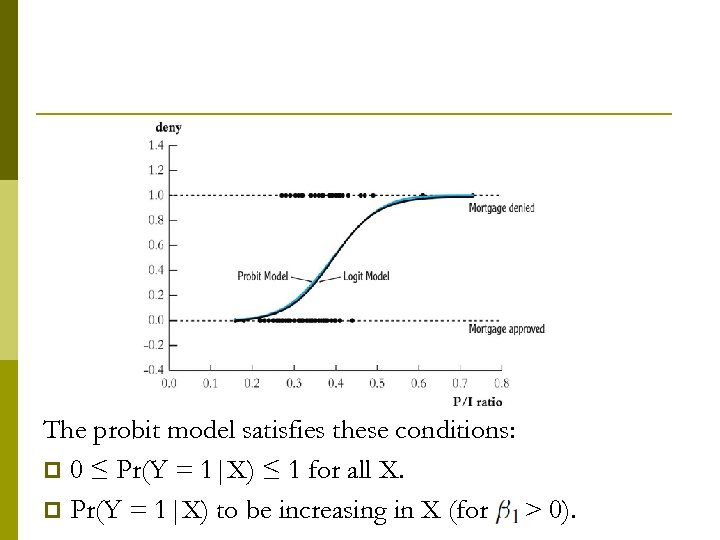The probit model satisfies these conditions: p 0 ≤ Pr(Y = 1|X) ≤ 1 for all X. p Pr(Y = 1|X) to be increasing in X (for > 0).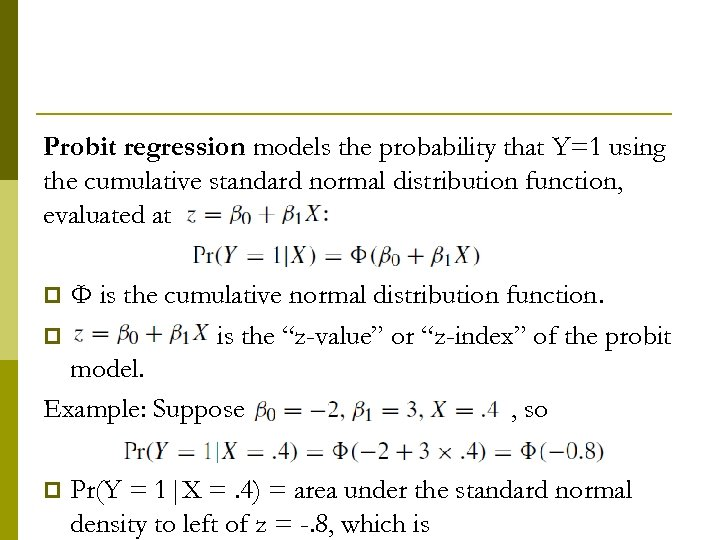Probit regression models the probability that Y=1 using the cumulative standard normal distribution function, evaluated at Φ is the cumulative normal distribution function. p is the “z-value” or “z-index” of the probit model. Example: Suppose , so p p Pr(Y = 1|X =. 4) = area under the standard normal density to left of z = -. 8, which is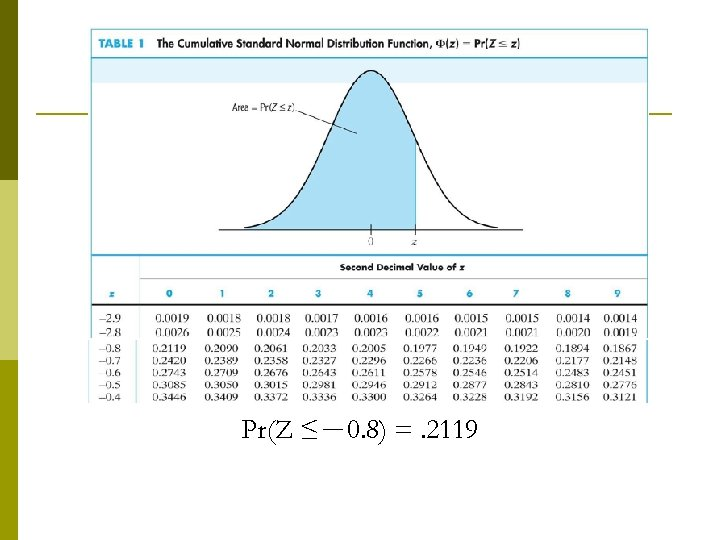Pr(Z ≤－0. 8) =. 2119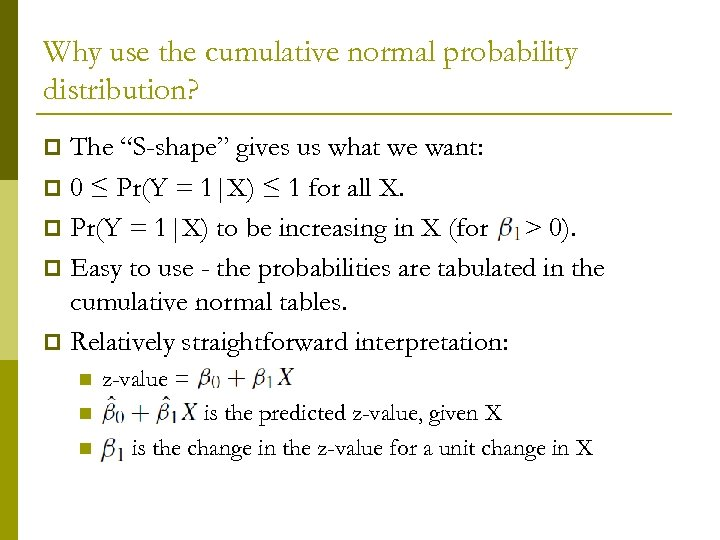Why use the cumulative normal probability distribution? The “S-shape” gives us what we want: p 0 ≤ Pr(Y = 1|X) ≤ 1 for all X. p Pr(Y = 1|X) to be increasing in X (for > 0). p Easy to use - the probabilities are tabulated in the cumulative normal tables. p Relatively straightforward interpretation: p n n n z-value = is the predicted z-value, given X is the change in the z-value for a unit change in X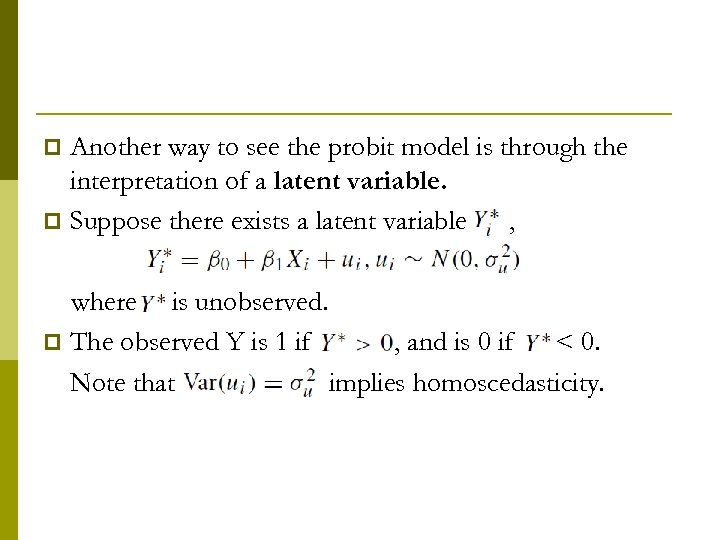Another way to see the probit model is through the interpretation of a latent variable. p Suppose there exists a latent variable , p where is unobserved. p The observed Y is 1 if , and is 0 if < 0. Note that implies homoscedasticity.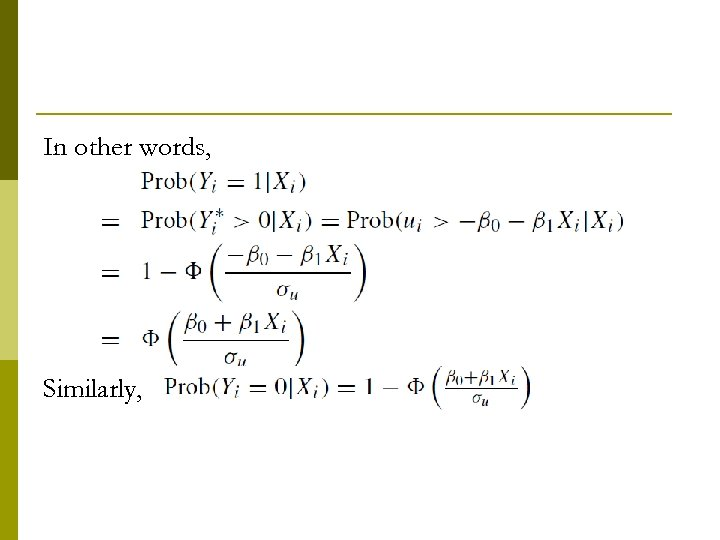In other words, Similarly,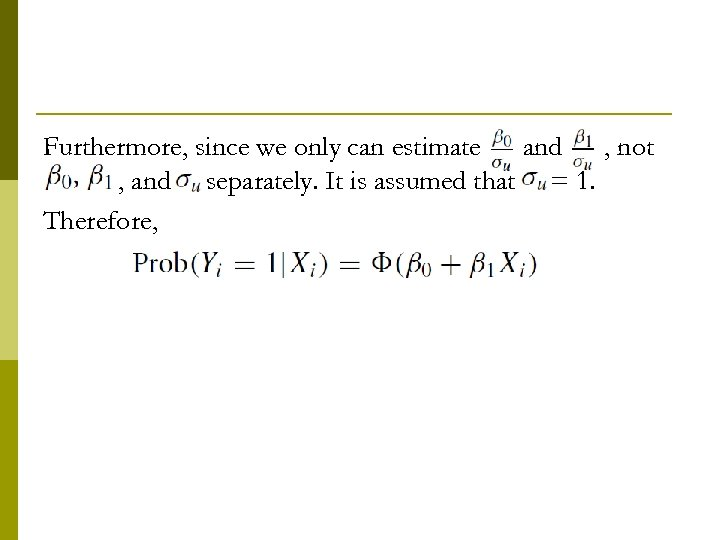Furthermore, since we only can estimate and , not 0 , and separately. It is assumed that = 1. Therefore,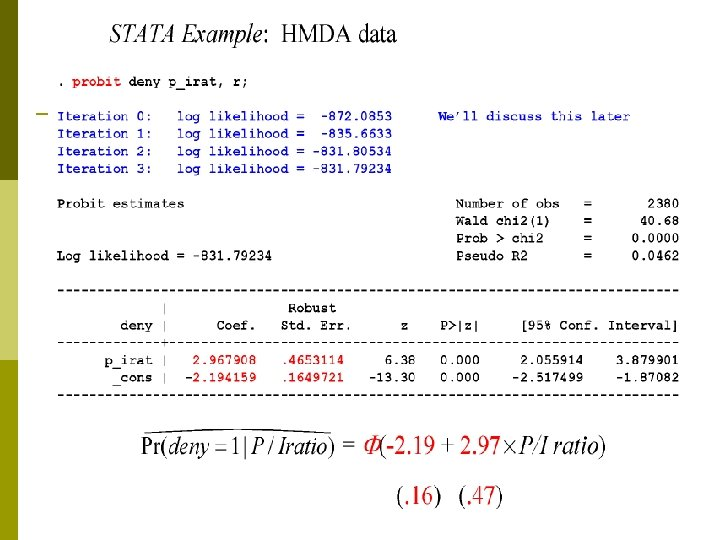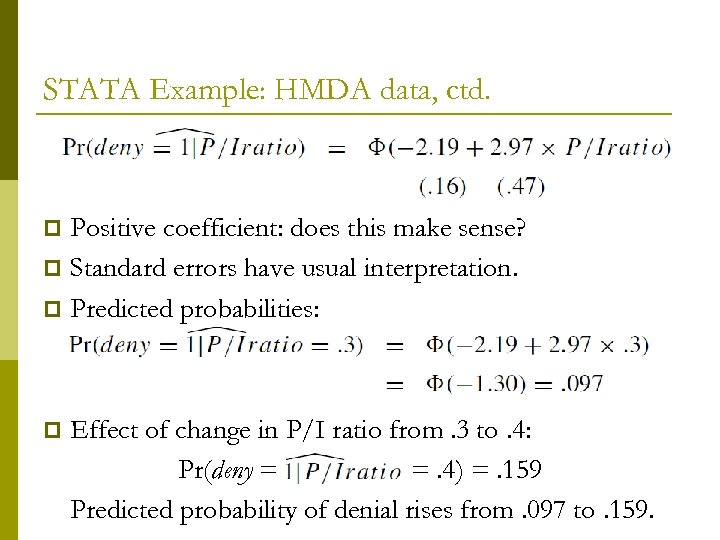STATA Example: HMDA data, ctd. Positive coefficient: does this make sense? p Standard errors have usual interpretation. p Predicted probabilities: p p Effect of change in P/I ratio from. 3 to. 4: Pr(deny = =. 4) =. 159 Predicted probability of denial rises from. 097 to. 159.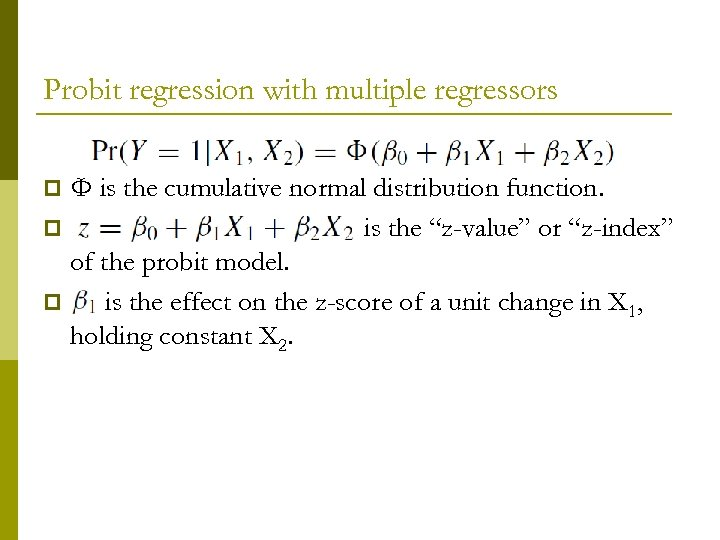Probit regression with multiple regressors Φ is the cumulative normal distribution function. p is the “z-value” or “z-index” of the probit model. p is the effect on the z-score of a unit change in X 1, holding constant X 2. p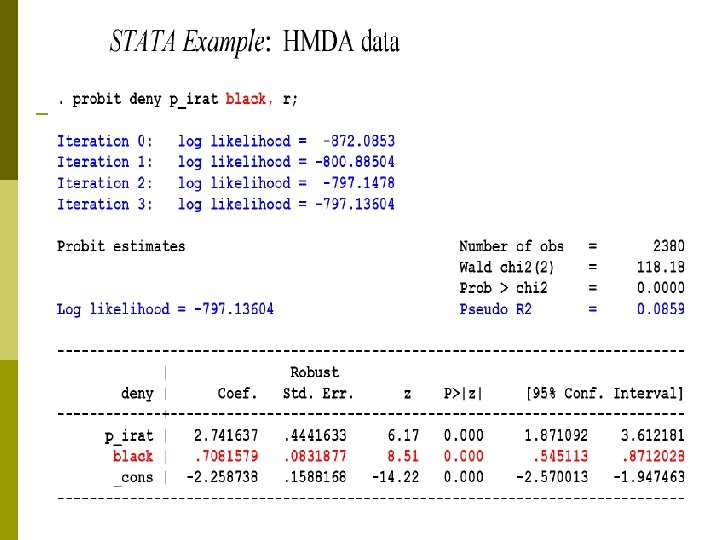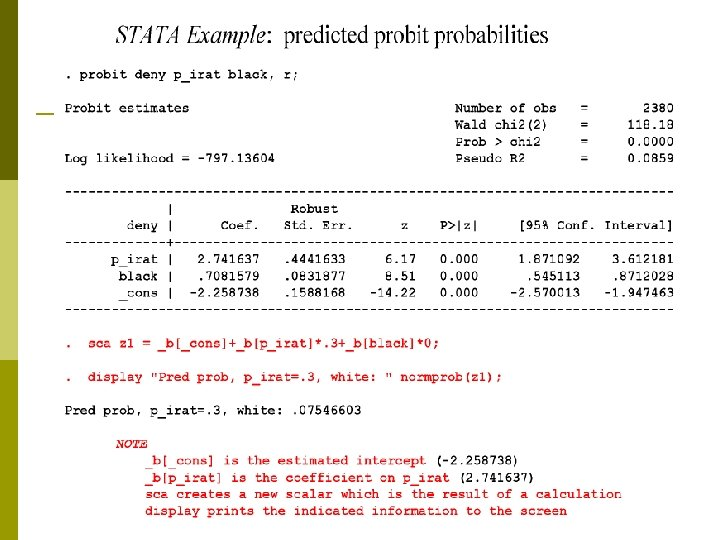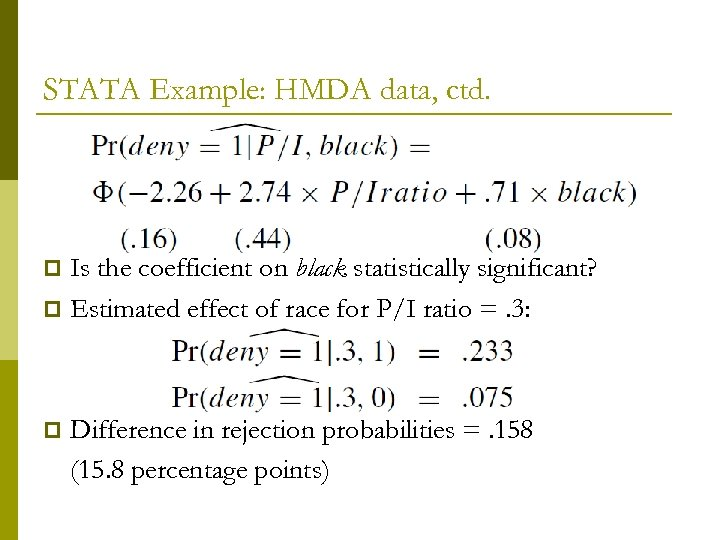STATA Example: HMDA data, ctd. Is the coefficient on black statistically significant? p Estimated effect of race for P/I ratio =. 3: p p Difference in rejection probabilities =. 158 (15. 8 percentage points)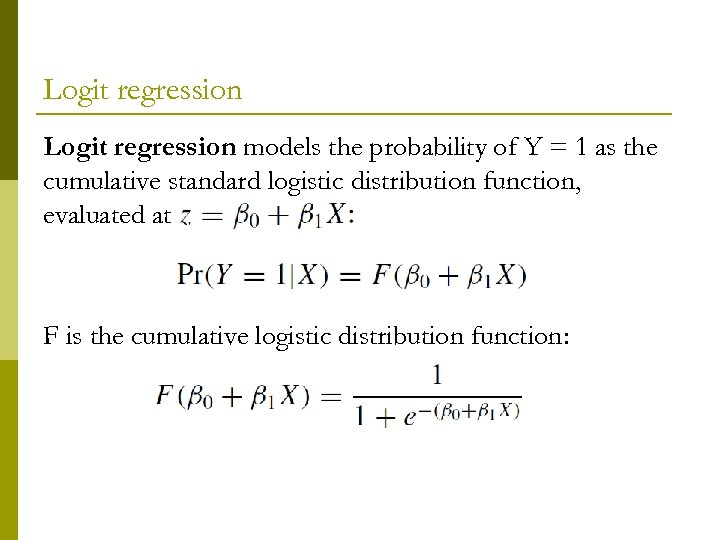Logit regression models the probability of Y = 1 as the cumulative standard logistic distribution function, evaluated at F is the cumulative logistic distribution function: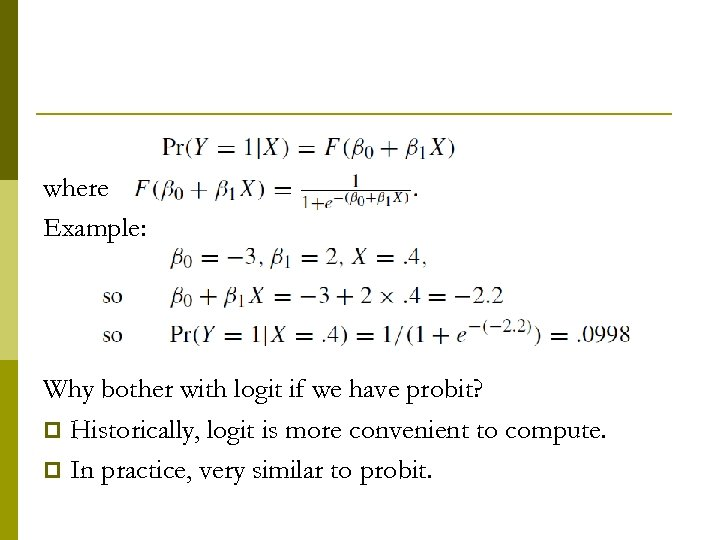where Example: Why bother with logit if we have probit? p Historically, logit is more convenient to compute. p In practice, very similar to probit.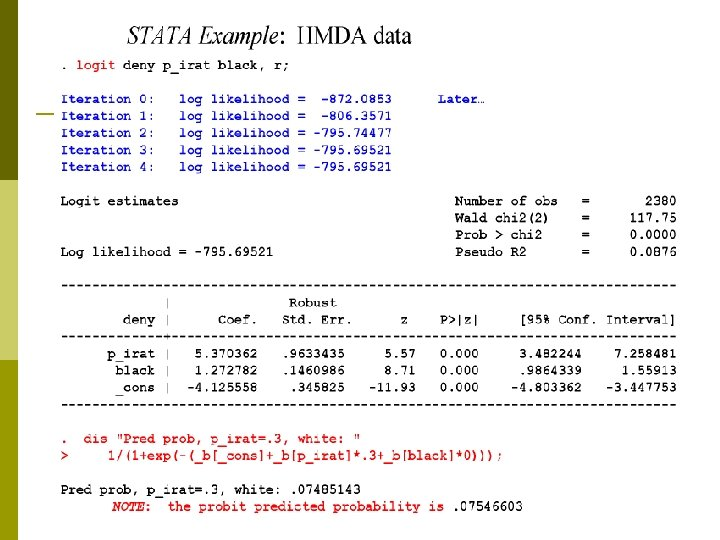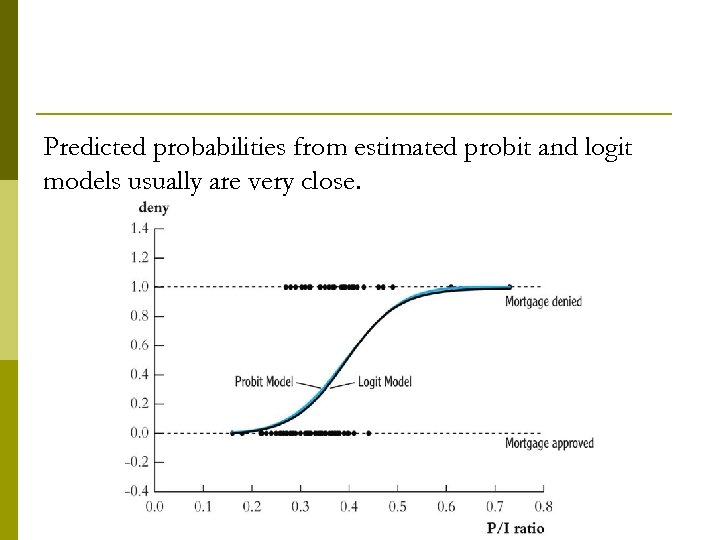Predicted probabilities from estimated probit and logit models usually are very close.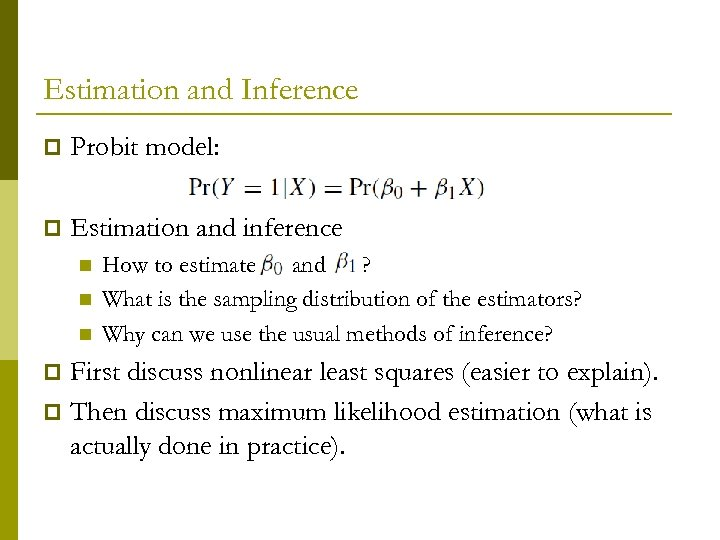Estimation and Inference p Probit model: p Estimation and inference n n n How to estimate and ? What is the sampling distribution of the estimators? Why can we use the usual methods of inference? First discuss nonlinear least squares (easier to explain). p Then discuss maximum likelihood estimation (what is actually done in practice). p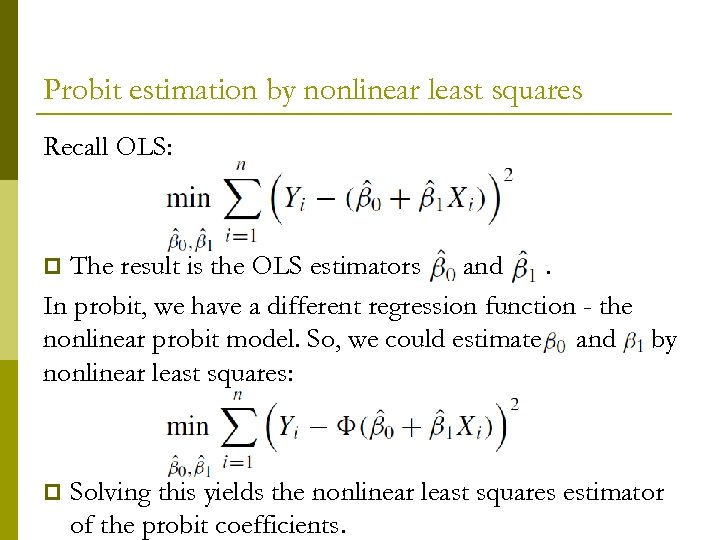Probit estimation by nonlinear least squares Recall OLS: The result is the OLS estimators and. In probit, we have a different regression function - the nonlinear probit model. So, we could estimate and by nonlinear least squares: p p Solving this yields the nonlinear least squares estimator of the probit coefficients.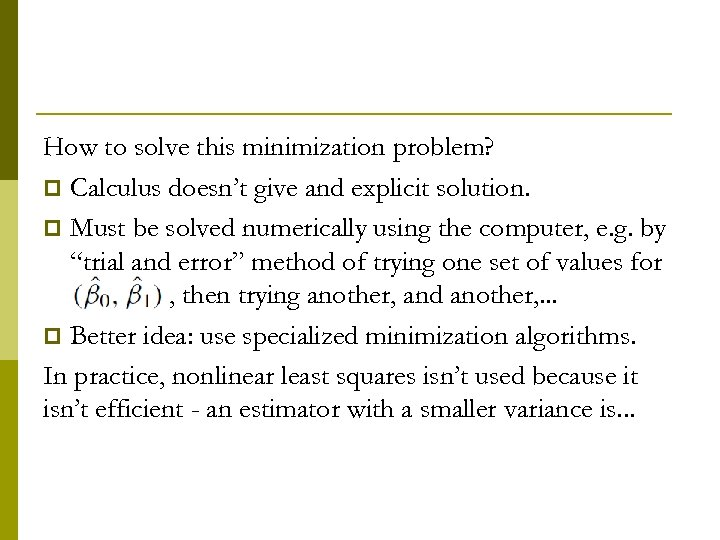How to solve this minimization problem? p Calculus doesn’t give and explicit solution. p Must be solved numerically using the computer, e. g. by “trial and error” method of trying one set of values for O , then trying another, and another, . . . p Better idea: use specialized minimization algorithms. In practice, nonlinear least squares isn’t used because it isn’t efficient - an estimator with a smaller variance is. . .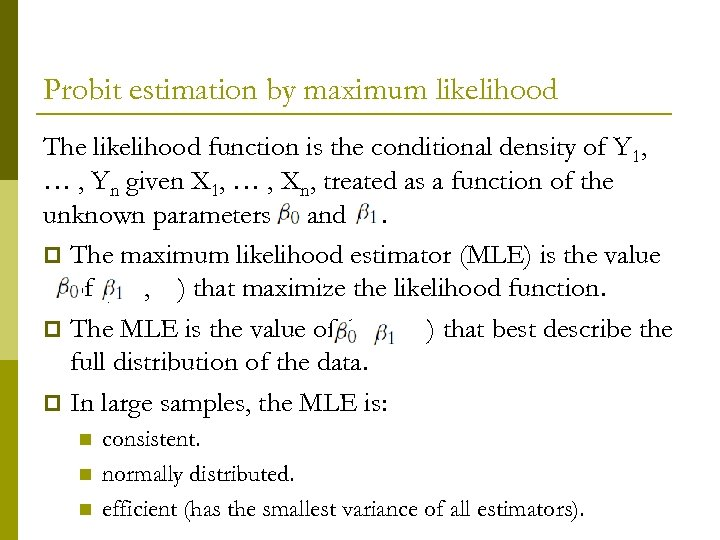Probit estimation by maximum likelihood The likelihood function is the conditional density of Y 1, … , Yn given X 1, … , Xn, treated as a function of the unknown parameters and. p The maximum likelihood estimator (MLE) is the value of ( , ) that maximize the likelihood function. p The MLE is the value of ( , ) that best describe the full distribution of the data. p In large samples, the MLE is: n n n consistent. normally distributed. efficient (has the smallest variance of all estimators).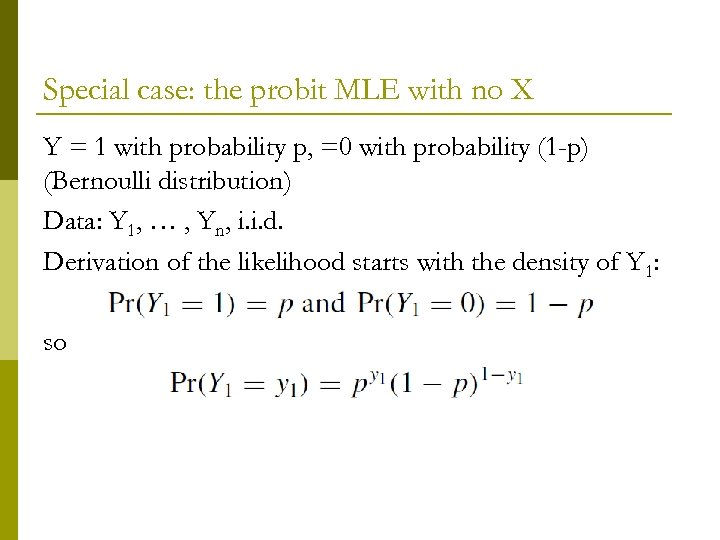Special case: the probit MLE with no X Y = 1 with probability p, =0 with probability (1 -p) (Bernoulli distribution) Data: Y 1, … , Yn, i. i. d. Derivation of the likelihood starts with the density of Y 1: so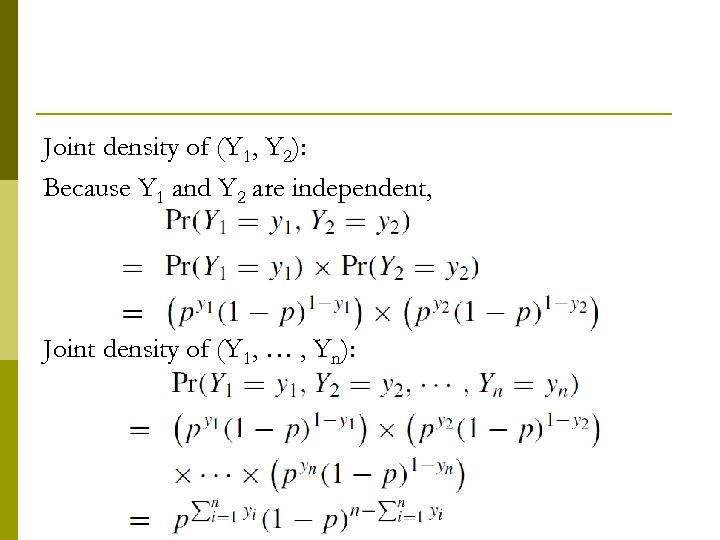Joint density of (Y 1, Y 2): Because Y 1 and Y 2 are independent, Joint density of (Y 1, … , Yn):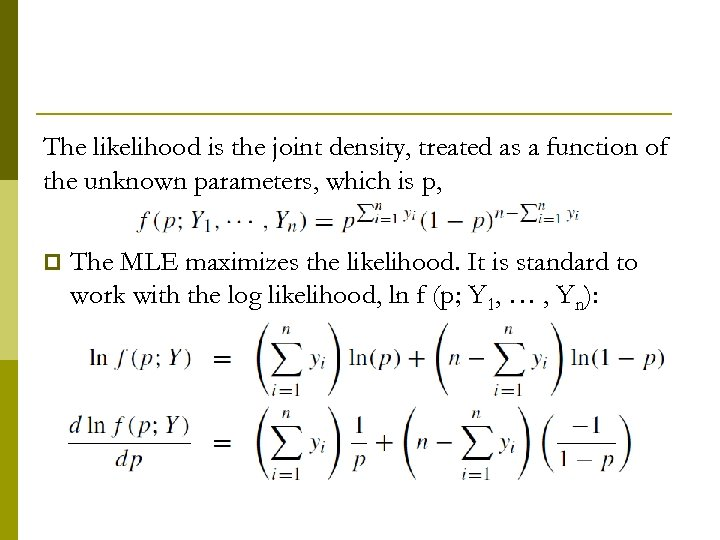The likelihood is the joint density, treated as a function of the unknown parameters, which is p, p The MLE maximizes the likelihood. It is standard to work with the log likelihood, ln f (p; Y 1, … , Yn):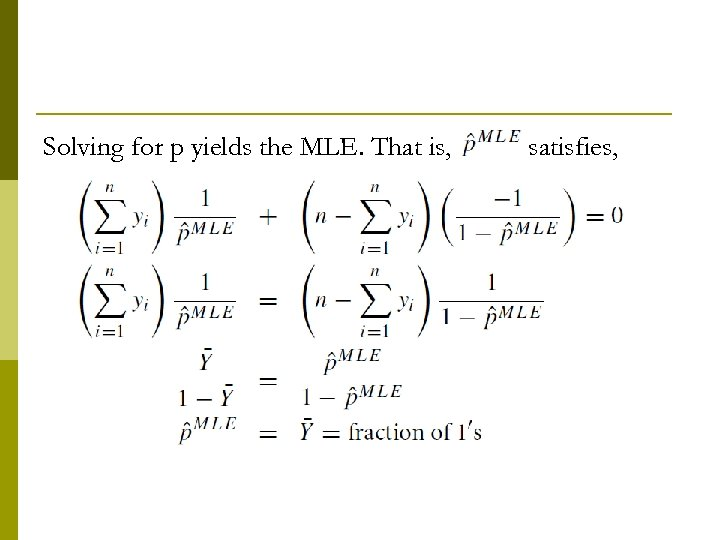Solving for p yields the MLE. That is, satisfies,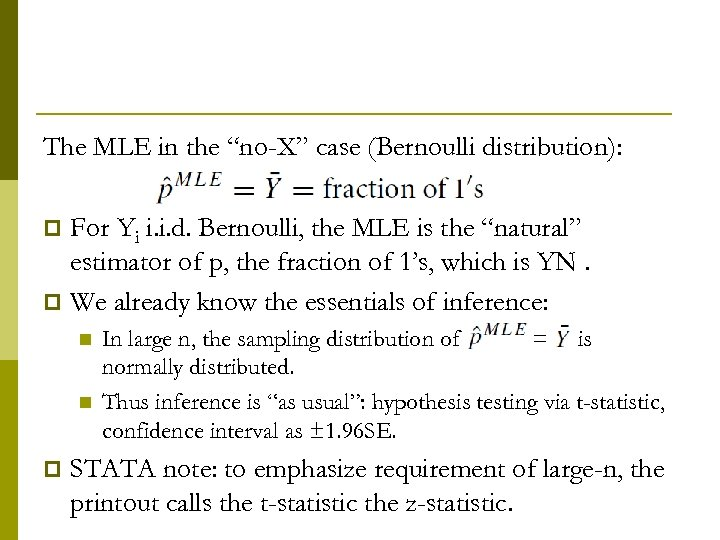The MLE in the “no-X” case (Bernoulli distribution): For Yi i. i. d. Bernoulli, the MLE is the “natural” estimator of p, the fraction of 1’s, which is YN. p We already know the essentials of inference: p n n p In large n, the sampling distribution of = is normally distributed. Thus inference is “as usual”: hypothesis testing via t-statistic, confidence interval as ± 1. 96 SE. STATA note: to emphasize requirement of large-n, the printout calls the t-statistic the z-statistic.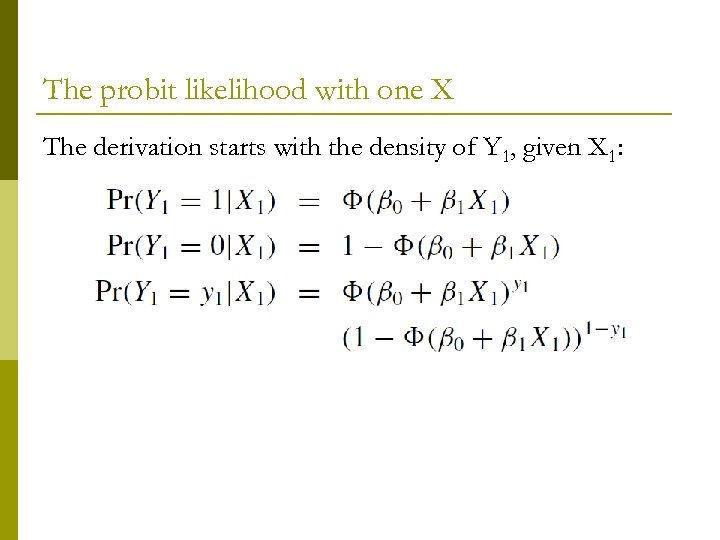The probit likelihood with one X The derivation starts with the density of Y 1, given X 1: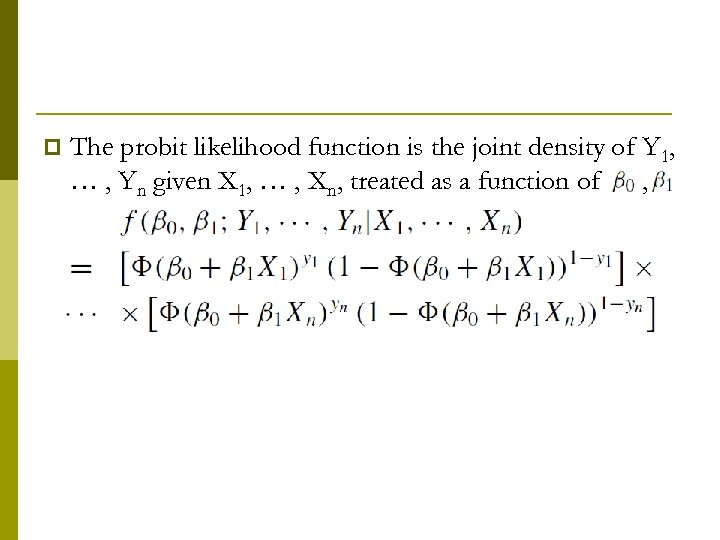p The probit likelihood function is the joint density of Y 1, … , Yn given X 1, … , Xn, treated as a function of ,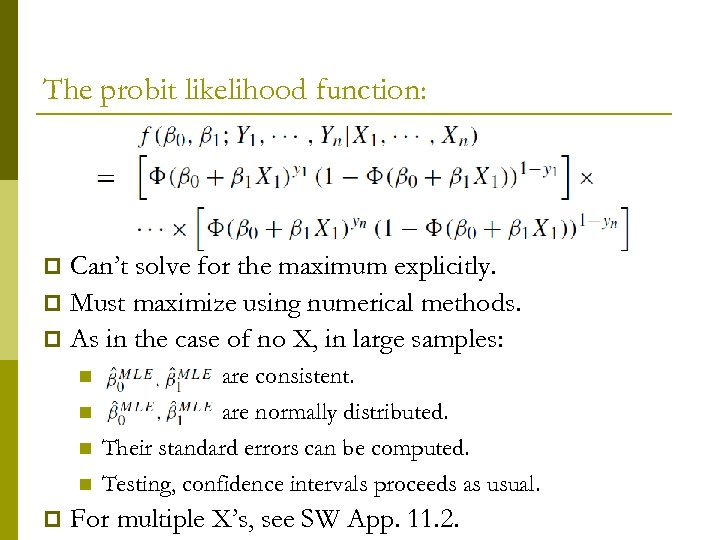The probit likelihood function: Can’t solve for the maximum explicitly. p Must maximize using numerical methods. p As in the case of no X, in large samples: p n n p are consistent. are normally distributed. Their standard errors can be computed. Testing, confidence intervals proceeds as usual. For multiple X’s, see SW App. 11. 2.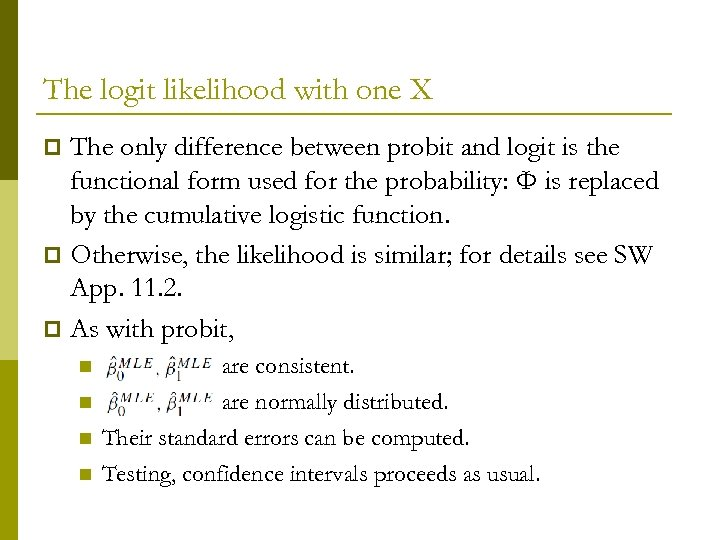The logit likelihood with one X The only difference between probit and logit is the functional form used for the probability: Φ is replaced by the cumulative logistic function. p Otherwise, the likelihood is similar; for details see SW App. 11. 2. p As with probit, p n n are consistent. are normally distributed. Their standard errors can be computed. Testing, confidence intervals proceeds as usual.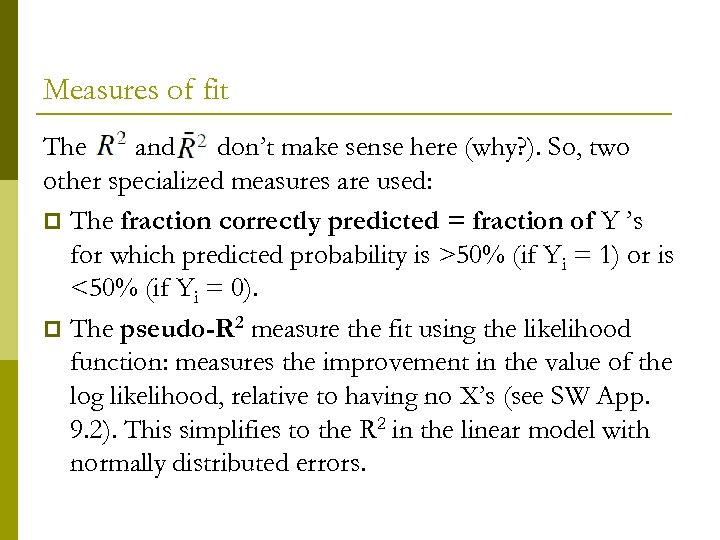Measures of fit The and don’t make sense here (why? ). So, two other specialized measures are used: p The fraction correctly predicted = fraction of Y ’s for which predicted probability is >50% (if Yi = 1) or is <50% (if Yi = 0). p The pseudo-R 2 measure the fit using the likelihood function: measures the improvement in the value of the log likelihood, relative to having no X’s (see SW App. 9. 2). This simplifies to the R 2 in the linear model with normally distributed errors.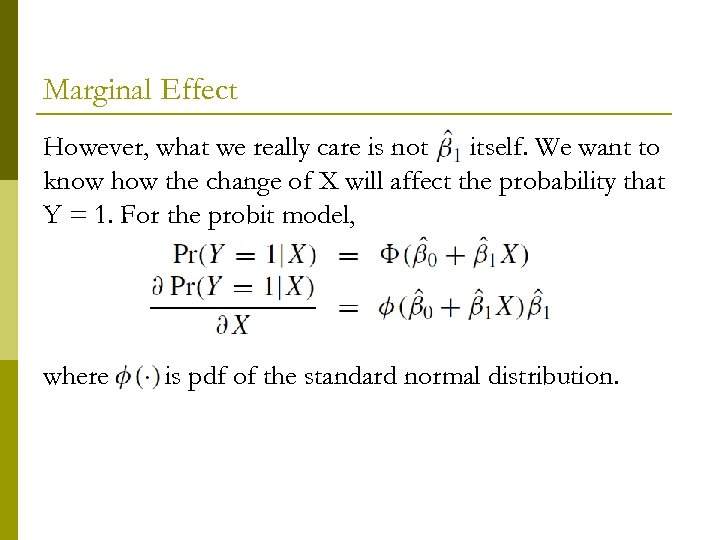Marginal Effect However, what we really care is not itself. We want to know how the change of X will affect the probability that Y = 1. For the probit model, where is pdf of the standard normal distribution.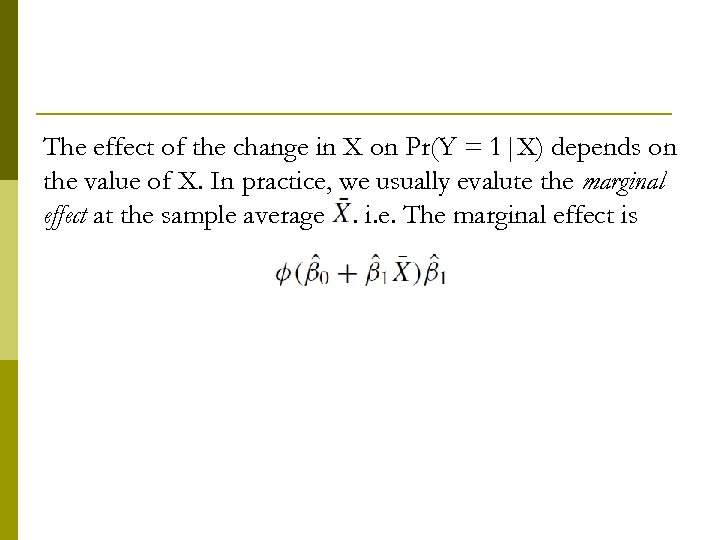The effect of the change in X on Pr(Y = 1|X) depends on the value of X. In practice, we usually evalute the marginal effect at the sample average. i. e. The marginal effect is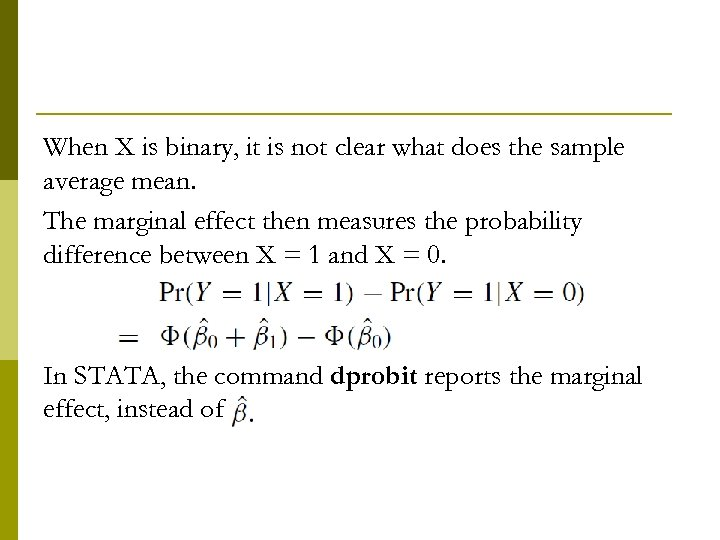When X is binary, it is not clear what does the sample average mean. The marginal effect then measures the probability difference between X = 1 and X = 0. In STATA, the command dprobit reports the marginal effect, instead of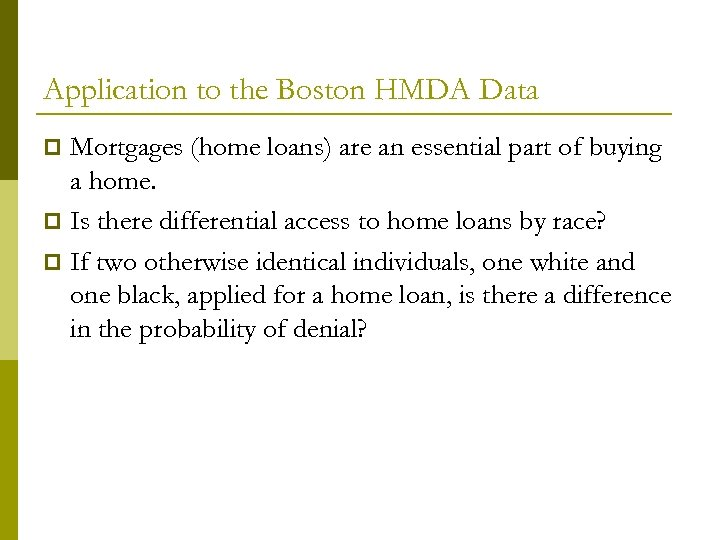Application to the Boston HMDA Data Mortgages (home loans) are an essential part of buying a home. p Is there differential access to home loans by race? p If two otherwise identical individuals, one white and one black, applied for a home loan, is there a difference in the probability of denial? p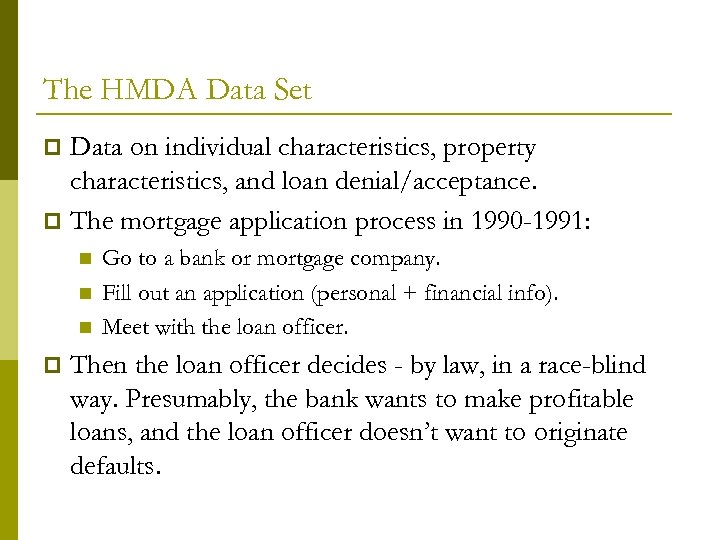The HMDA Data Set Data on individual characteristics, property characteristics, and loan denial/acceptance. p The mortgage application process in 1990 -1991: p n n n p Go to a bank or mortgage company. Fill out an application (personal + financial info). Meet with the loan officer. Then the loan officer decides - by law, in a race-blind way. Presumably, the bank wants to make profitable loans, and the loan officer doesn’t want to originate defaults.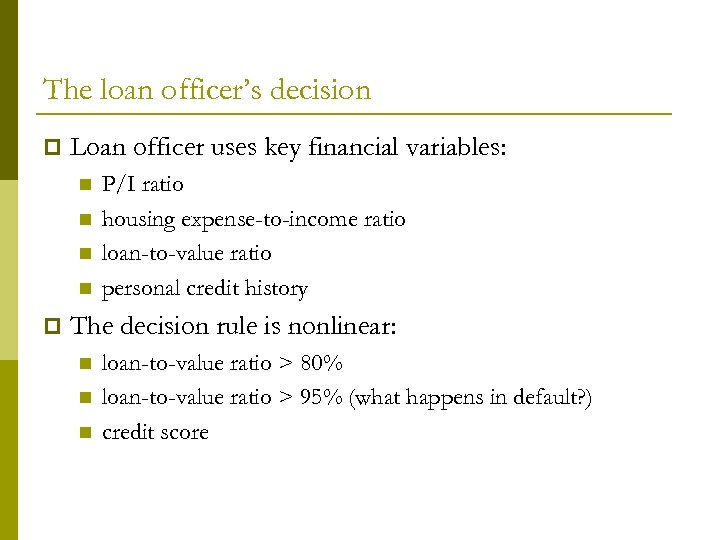The loan officer’s decision p Loan officer uses key financial variables: n n p P/I ratio housing expense-to-income ratio loan-to-value ratio personal credit history The decision rule is nonlinear: n n n loan-to-value ratio > 80% loan-to-value ratio > 95% (what happens in default? ) credit score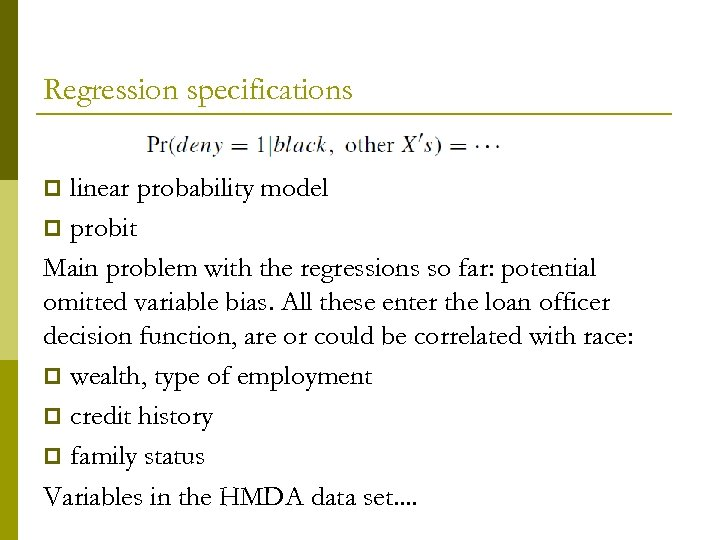Regression specifications linear probability model p probit Main problem with the regressions so far: potential omitted variable bias. All these enter the loan officer decision function, are or could be correlated with race: p wealth, type of employment p credit history p family status Variables in the HMDA data set. . p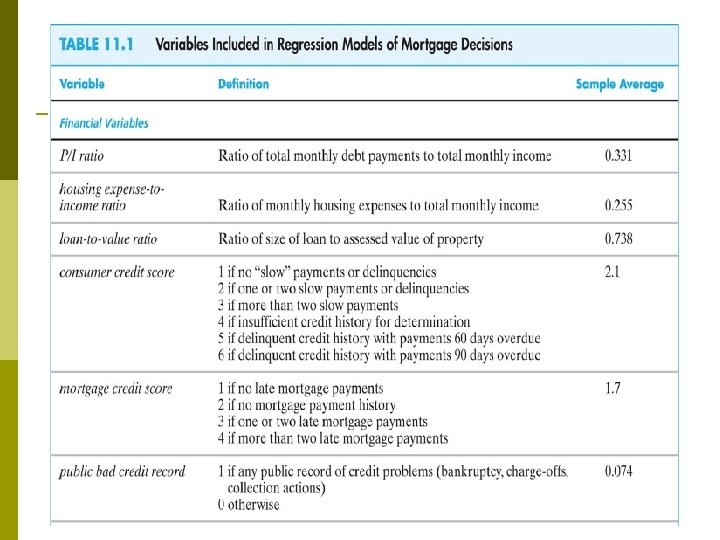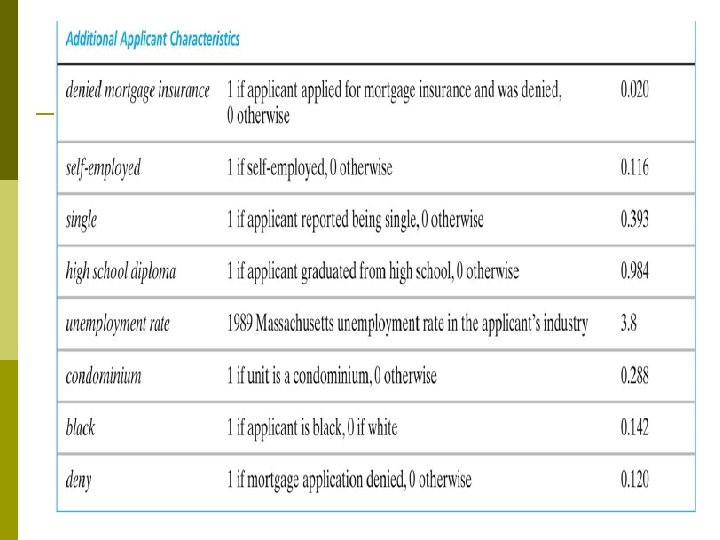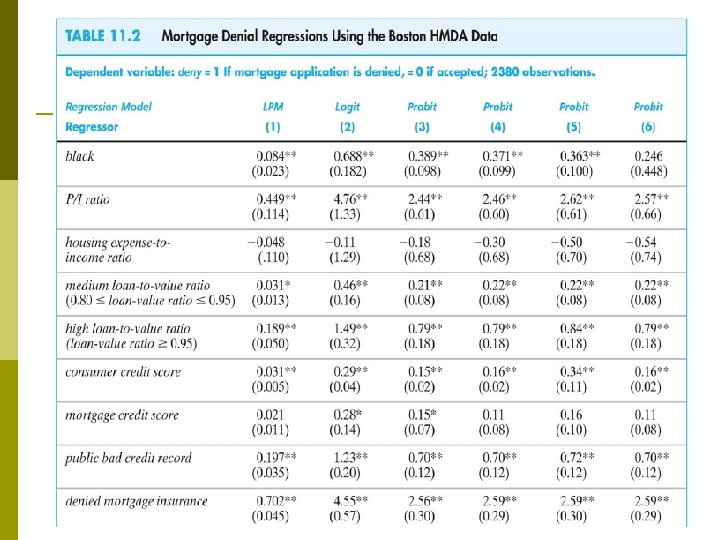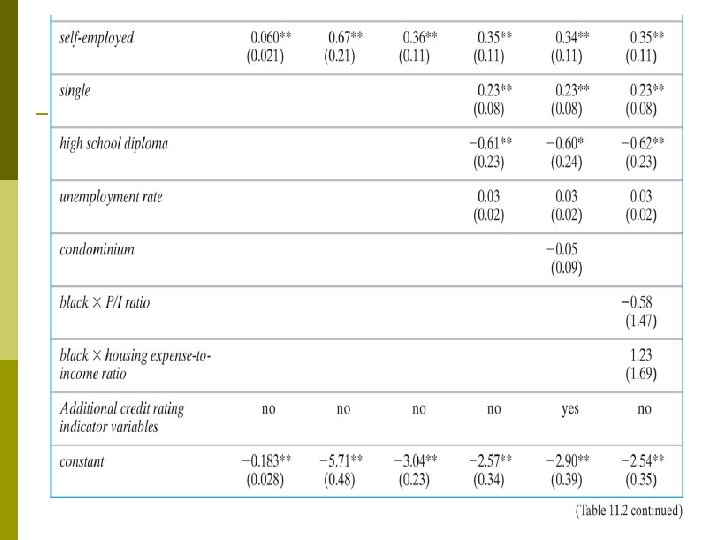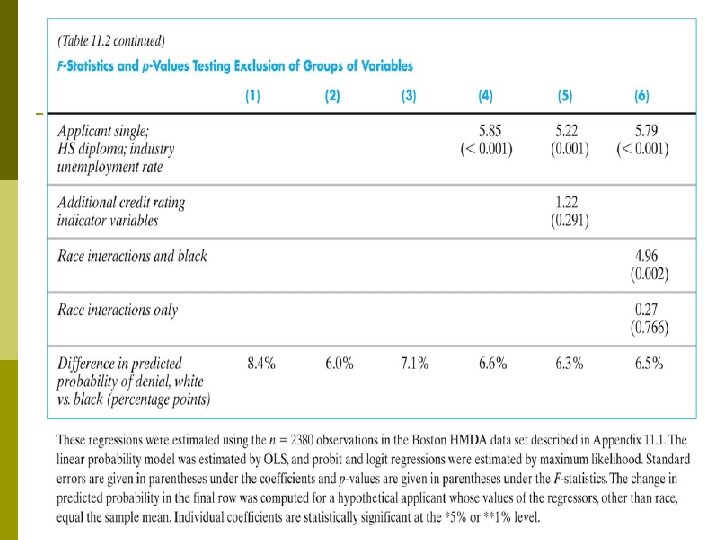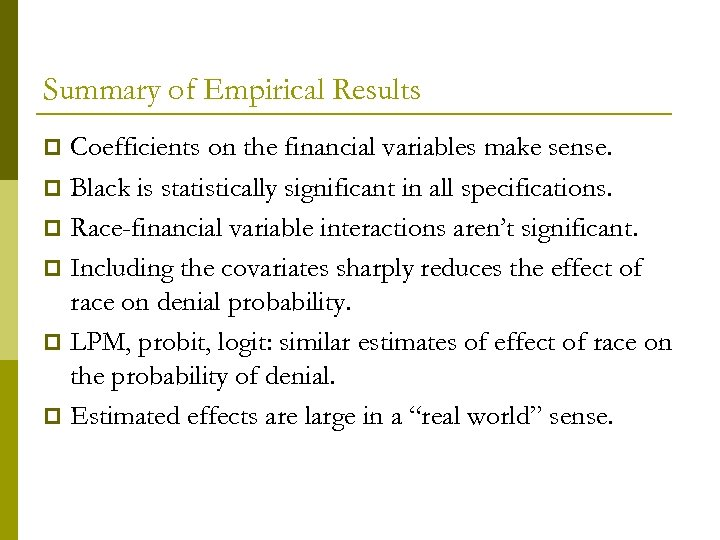Summary of Empirical Results Coefficients on the financial variables make sense. p Black is statistically significant in all specifications. p Race-financial variable interactions aren’t significant. p Including the covariates sharply reduces the effect of race on denial probability. p LPM, probit, logit: similar estimates of effect of race on the probability of denial. p Estimated effects are large in a “real world” sense. p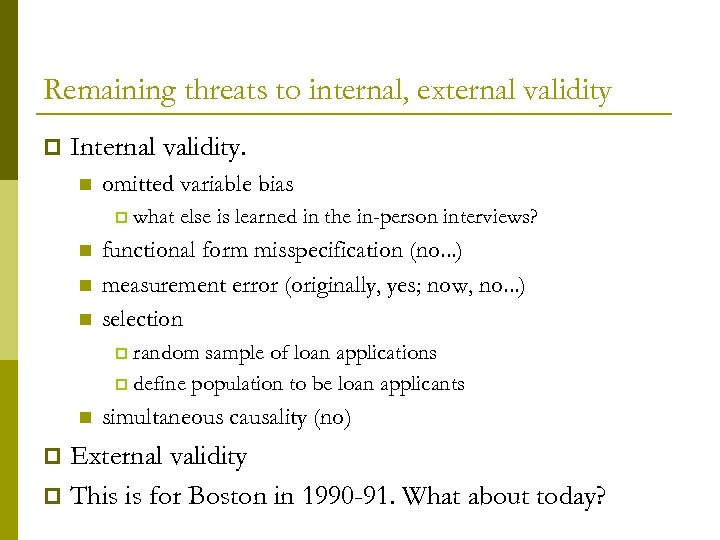Remaining threats to internal, external validity p Internal validity. n omitted variable bias p n n n what else is learned in the in-person interviews? functional form misspecification (no. . . ) measurement error (originally, yes; now, no. . . ) selection random sample of loan applications p define population to be loan applicants p n simultaneous causality (no) External validity p This is for Boston in 1990 -91. What about today? p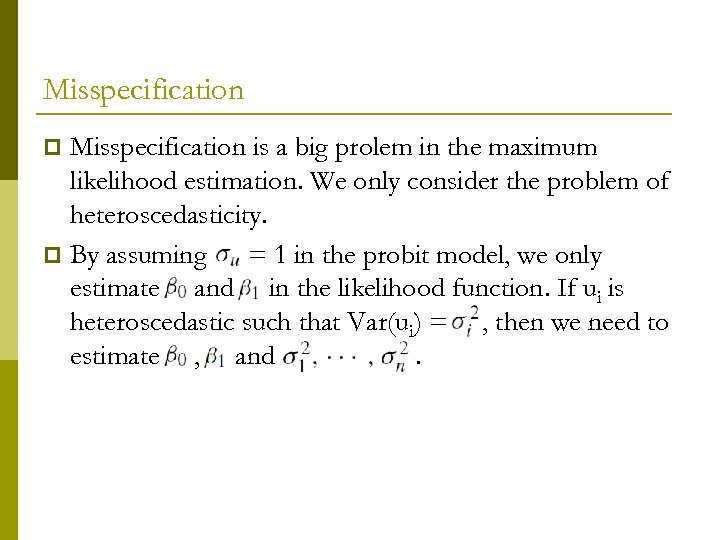Misspecification is a big prolem in the maximum likelihood estimation. We only consider the problem of heteroscedasticity. p By assuming = 1 in the probit model, we only estimate and in the likelihood function. If ui is heteroscedastic such that Var(ui) = , then we need to estimate , and. p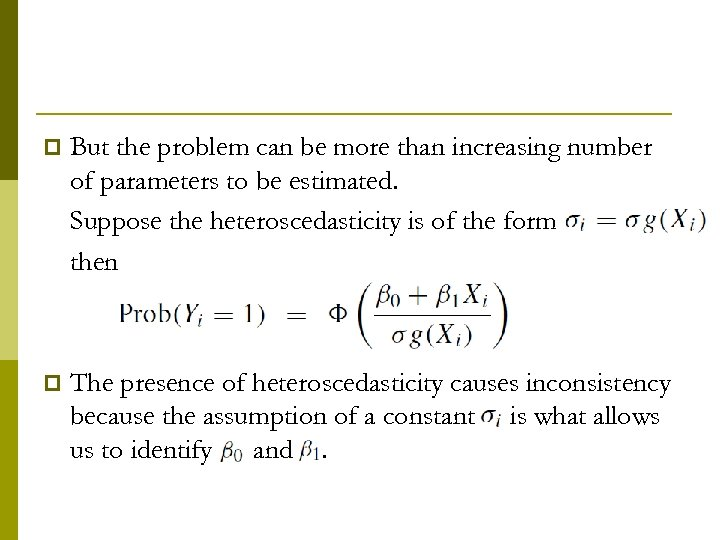p But the problem can be more than increasing number of parameters to be estimated. Suppose the heteroscedasticity is of the form then p The presence of heteroscedasticity causes inconsistency because the assumption of a constant is what allows us to identify 0 and.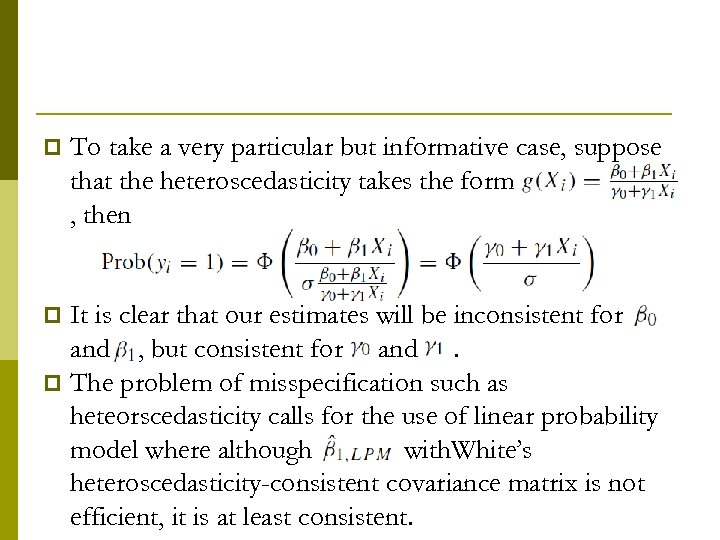p To take a very particular but informative case, suppose that the heteroscedasticity takes the form , then It is clear that our estimates will be inconsistent for and , but consistent for and. p The problem of misspecification such as heteorscedasticity calls for the use of linear probability model where although with. White’s heteroscedasticity-consistent covariance matrix is not efficient, it is at least consistent. p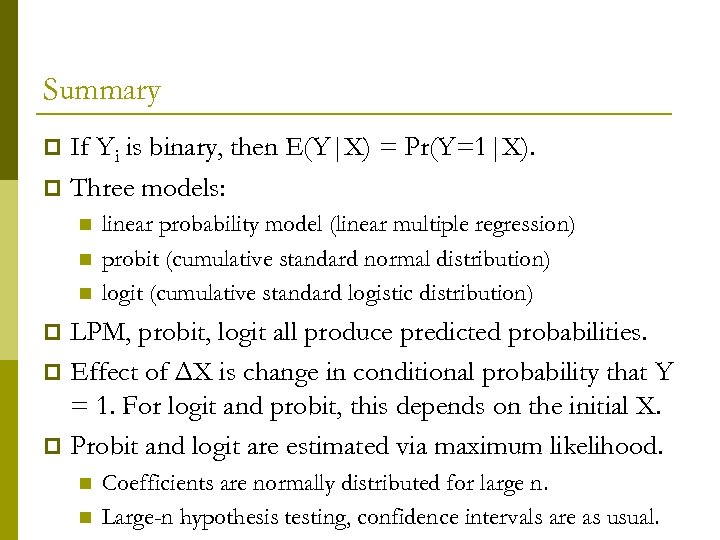Summary If Yi is binary, then E(Y|X) = Pr(Y=1|X). p Three models: p n n n linear probability model (linear multiple regression) probit (cumulative standard normal distribution) logit (cumulative standard logistic distribution) LPM, probit, logit all produce predicted probabilities. p Effect of ΔX is change in conditional probability that Y = 1. For logit and probit, this depends on the initial X. p Probit and logit are estimated via maximum likelihood. p n n Coefficients are normally distributed for large n. Large-n hypothesis testing, confidence intervals are as usual.